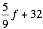# Sample Midterm 1

1. Which of the following Python identifiers are legal variable names? For those that are not, say why not.
1. x
2. Celsius
3. spam3
4. <hello>
5. question?
2. What is the data type (int, long int, float, string, list) of the following:
1. 3
2. 3.0
3. 3L
4. "hello"
5. 'x'
6. [ 1, 2, 3 ]
3. Write the following formulas in Python:
1.2. 7 × 9
3. 42
4. What does the following program print?
for i in "hello":
print i, "-->", chr(ord(o)+1);
5. What does the following program print?
for i in [1, 7, 4]:
x = (-1) ** i * i;
print i, x;
6. When I ran the following program:
x = input("Enter a number: ");
y = 1.0 / x;
print "The reciprocal of", x, "is", y;
I got the following error message:
Traceback (most recent call last):
File "test.py", line 2, in <module>
y = 1 / x;
ZeroDivisionError: integer division or modulo by zero
What caused the error? Please be specific.